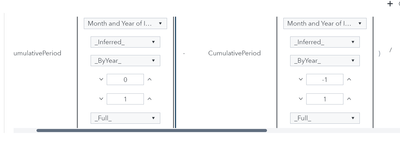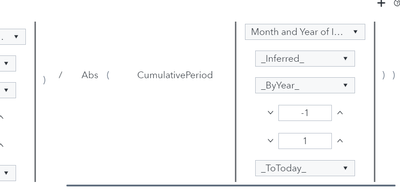## How to calculate year to date growth based on fiscal year

Dear All,

I am using SAS Viya 4.0 2022 LTS version.

I am facing difficulty in calculating the YTD Growth based on fiscal Year.

Fiscal Year should start from 1st April.

Case 1 : If an user wants to check  YTD growth value for 10th August 2022 then the calculation should be done taking 1st April 2022.

Case 2 :  If an user wants to check  YTD growth value for 23rd March 2022 then the calculation should be done taking 1st April 2021 as the starting period.

Could you please tell me the exact values I need to put in the calculated field or the steps I need to follow to achieve the same.

7 REPLIES 7Ksharp
Super User

## Re: How to calculate year to date growth based on fiscal year

``````data have;
input have anydtdte32. ;
want=intnx('year.4',have,0);
format have want worddatx.;
cards;
10 August 2022
23 March 2022
23 March 2023
;
``````

## Re: How to calculate year to date growth based on fiscal year

Hi @Ksharp,

Thanks for looking forward to my problem.
But, I need to do in SAS Visual Analytics and I do not have any exposure to code in SAS Enterprise Guide .

FYI, the Case 1 and Case 2 which I mentioned earlier were just examples , I need to do the YTD calculation for a very wide range of duration.

Could you please let me know how it is done in SAS VA?Ksharp
Super User

## Re: How to calculate year to date growth based on fiscal year

Sorry. I have barely experience with SAS VA.

## Re: How to calculate year to date growth based on fiscal year

Dear All,

I have to calculate YTD Growth based on fiscal year in SAS Visual Analytics, with April as the staring period and March as the end.

Could you please let me know how it can be done on some measure 'X' and date field 'Y'.(The above images are from the New Calculation > Year To Date Growth)

What should be the inner and outer interval in the above attached images(kindly ignore the values that appear in the images) for fiscal year with starting period as April and end period as March?Sam_SAS
SAS Super FREQ

## Re: How to calculate year to date growth based on fiscal year

Hello,

You are on the right track! If you edit the expression that is generated from the YTD Growth quick calculation, I think you should get what you need.

The second-to-last parameter specifies which month of the year is the start of the year. So if you change that 1 to a 4, in each instance of the CumulativePeriod () operator, that should change the calculation to use April as the year start.

Let us know if that yields the result you wanted.

Thanks,

Sam

## Re: How to calculate year to date growth based on fiscal year

Hi Sam,

There will be issue here if I change the value to 4 as cumulative sum will be calculated and I need the second field to have fixed value.

I want second parameter to have fixed value for a particular year and it should be having value of the month of April as the fiscal year starts with April.

For Eg,
In April 2021, i have ytd_measure1 =10

In October 2021 , i have ytd_measure1= 90

In March 2022 , i have ytd_measure1=190

So YTD Growth in October 2021 = (90-10)/10 *100%=800%

YTD Growth in March 2022= (190-10)/10 * 100% =1800%Sam_SAS
SAS Super FREQ

## Re: How to calculate year to date growth based on fiscal year

I have been experimenting with this, and I think what you want might not actually be possible using the VA expression editor.

The "Year to Date  Growth" calculation is actually to compare the Year-over-year growth of the YTD sum. It does not compare to the value of the first month in the current fiscal year.

The following will give you the YTD value based on April as the fiscal year start:

CumulativePeriod(_Sum_, 'Product Sale'n, IgnoreAllTimeFrameFilters, 'Transaction Month'n, Inferred, Inferred, 0, 4, Full)

But I don't think there is a good way to get the YTD value as a percentage from month to month. You could pre-calculate these values as part of your data preparation.

The expression builder in general and handling of date values specifically are focuses for the VA dev team and we hope to expand on the functionality in the future.

Sam
Discussion stats
• 7 replies
• 528 views
• 0 likes
• 3 in conversation